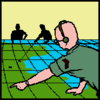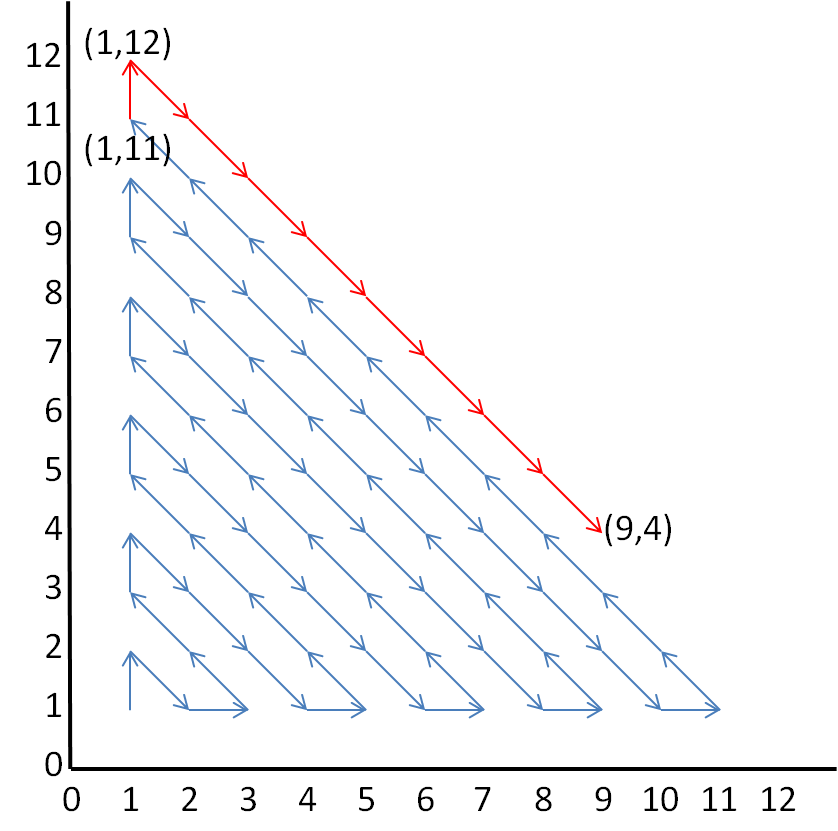#### You may also like### Coordinate Patterns

Charlie and Alison have been drawing patterns on coordinate grids. Can you picture where the patterns lead?### Going Places with Mathematicians

This article looks at the importance in mathematics of representing places and spaces mathematics. Many famous mathematicians have spent time working on problems that involve moving and mapping things.### Coordinates and Descartes

Have you ever wondered how maps are made? Or perhaps who first thought of the idea of designing maps? We're here to answer these questions for you.

# Route to Infinity

##### Age 11 to 14Challenge Level

After (18, 17), the route will visit (19, 16). Lots of people got this right - let's have a look at just a few of their solutions:

Tiberiu noticed:

I see that the route goes upwards through the points with coordinates that are equal (eg. 18,18) and downwards through the points with coordinates that are adjacent (eg. 18,17). Therefore the next coordinate is (19,16).

Lawrence, from Chircher's College, worked it out like this:

Looking at the diagram you can tell that if the x coordinate of the bottom right point on a line is even, the arrows in the line go down and right, otherwise, they go up and left. The point (18,17) is on the line with bottom right point (18+17-1,1) = (34,1). Because 34 is even, the arrows go down and right, so the next point is (19,16).

Alex, from Dixons City Academy, spotted the same thing, in a different way:

The numbers all lie on lines with equations x+y=n, where n is some integer. If n is odd, then the line begins at (1, n-1). These arrows point towards the bottom right. If n is even, the line begins at coordinate (n-1, 1) and the direction of the line is towards the top left. Notice that 18+17=35, so the coordinate (18, 17) is on the line x+y=35.

Segev, Adam, Yoav, Daniel and Jonathan from JFS answered the second part of our question:

There are 74 points visited before the route reaches the point (9,4). The number of points visited follows the triangle numbers.
Number of points visited up to (1,1): 1
Number of points visited up to (2,1): 3
Number of points visited up to (1,3): 6
Number of points visited up to (4,1): 10
etc.

Therefore the number of points visited up to (1,11) is 66.

It is worth noticing here that because $9+4=13$ is odd, the line points towards the top left, so we are starting at (1,11).

Then, we need to visit 8 points on the diagonal before reaching (9,4), which are to (1,12), (2,11), (3,10), (4,9), (5,8), (6,7), (7,6) and (8,5) before reaching (9,4).

This is a total of $66+8=74$ points visited before (9,4).

The diagram below shows the 66 steps in blue, and then these final steps in red. Although there are nine red steps marked, only eight are counted as it is the number before (9,4) that is requested.There were far too many great solutions to list here - thanks to everyone who submitted one!